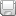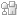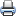Home > Articles > Cisco Certification > CCNA Routing and Switching > Fundamentals of IP for the CCNA INTRO Exam #640-821

# Fundamentals of IP for the CCNA INTRO Exam #640-821SaveDiggDel.icio.usPrint

### Chapter Description

Learn about the core concepts behind OSI Layer 3 and the main Layer 3 protocol used by TCP/IP to help you on the CCNA INTRO Exam.

## Q&A

As mentioned in the introduction, you have two choices for review questions. The questions that follow give you a bigger challenge than the exam itself by using an open-ended question format. By reviewing now with this more difficult question format, you can exercise your memory better and prove your conceptual and factual knowledge of this chapter. The answers to these questions are found in Appendix A.

For more practice with exam-like question formats, including questions using a router simulator and multiple-choice questions, use the exam engine on the CD.

1. What are the two main functions of each OSI Layer 3–equivalent protocol?

2. Assume that PC1 sends data to PC2, and PC2 is separated from PC1 by at least one router. Are the IP addresses of the PCs in the same IP subnet? Explain your answer.

3. Assume that PC1 sends data to PC2, and PC2 is not separated from PC1 by at least one router. Are the IP, addresses of the PCs in the same IP subnet? Explain your answer.

4. How many bits are present in an IP address?

5. How many bits are present in an IPX address?

6. How many bits are present in an AppleTalk address?

7. Name the two main parts of an IPX address. Which part identifies which group this address is a member of?

8. Name the two main parts of an IP address. Which part identifies which group this address is a member of?

9. PC1 sends data to PC2 using TCP/IP. Three routers separate PC1 and PC2. Explain why the statement "PC1 sends an Ethernet frame to PC2" is true or false.

10. In IP addressing, how many octets are in 1 byte?

11. Describe the differences between a routed protocol and a routing protocol.

12. Name at least three routed protocols.

13. Name at least three IP routing protocols.

14. Imagine an IP host on an Ethernet, with a single router attached to the same segment. In which cases does an IP host choose to send a packet to this router instead of directly to the destination host, and how does this IP host know about that single router?

15. Name three items in an entry in any routing table.

16. Name the parts of an IP address when subnetting is used.

17. How many valid IP addresses exist in a Class A network? (You may refer to the formula if you do not know the exact number.)

18. How many valid IP addresses exist in a Class B network? (You may refer to the formula if you do not know the exact number.)

19. How many valid IP addresses exist in a Class C network? (You may refer to the formula if you do not know the exact number.)

20. What values can a Class A network have in the first octet?

21. What values can a Class B network have in the first octet?

22. What values can a Class C network have in the first octet?

23. When subnetting a Class B network, do you create the subnet field by taking bits from the network part of the address or the host part?

24. When subnetting a Class B network, using the entire third octet for the subnet part, describe the number of possible subnets created.

25. When subnetting a Class A network using the entire second octet for the subnet part, describe the number of hosts in each subnet.

26. When a router hears about multiple routes to the same subnet, how does it choose which route to use?

27. What is the primary purpose of a routing protocol?

28. True or false: "Routing protocols are required to learn routes of directly connected subnets."

29. Which IP routing protocols are Cisco proprietary?

30. List the similarities and differences between RARP and BOOTP.

31. List the similarities and differences between DHCP and BOOTP.

32. List the similarities and differences between ARP and DNS.